# Heat

In thermodynamics, heat is energy transferred from one object to another. Heat spontaneously flows from an object with a high temperature to an object with a lower temperature. The transfer of heat from an object, to another object with an equal or higher temperature, however, can happen only with the aid of a heat pump.

## Contents

Heat is transferred between objects by radiation, conduction, or convection. Temperature is used as a measure of the internal energy or enthalpy, that is the level of elementary motion giving rise to heat transfer. Heat can only be transferred between objects or areas within an object with different temperatures (as given by the zeroth law of thermodynamics), and then, in the absence of work, only in the direction of the colder body (as per the second law of thermodynamics). The temperature and state of a substance subject to heat transfer are determined by latent heat and heat capacity. A related term, thermal energy, is loosely defined as the energy in a body that increases with its temperature.

## Overview

Heat is the transfer of energy caused by temperature difference. The first law of thermodynamics states that the energy of a closed system is conserved. Therefore, to change the energy of a system, energy must be transferred to or from the system. Heat and work are the only two mechanisms by which energy can be transferred to or from a control mass. The unit for the amount of energy transferred by heat in International System of Units (SI) is the Joule—though the British Thermal Unit and calorie are still occasionally used in the United States—and the unit for the rate of heat transfer is the Watt (J/s).

Heat transfer is a path function (process quantity), as opposed to a point function (state quantity). Heat flows between systems that are not in thermal equilibrium with each other; it spontaneously flows from the areas of high temperature to areas of low temperature. When two bodies of different temperature come into thermal contact, they will exchange internal energy until their temperatures are equalized (thermal equilibrium). The term heat is used to describe the flow of energy. In the absence of work interactions, the heat that is transferred to an object ends up getting stored in the object in the form of internal energy.

Specific heat of a substance is the amount of energy that has to be transferred to or from one unit of mass or mole of a substance to change its temperature by one degree. Specific heat is a property, which means that it depends on the substance under consideration and its state as specified by its properties. Fuels, when burned, release much of the energy in the chemical bonds of their molecules. Upon changing from one phase to another, a pure substance releases or absorbs heat without its temperature changing. The amount of heat transfer during a phase change is known as latent heat and depends primarily on the substance and its state.

## History

The first recorded to have put forward a theory on heat was the Greek philosopher Heraclitus, who lived around 500 B.C.E. in the city of Ephesus in Ionia, Asia Minor. He became famous as the "flux and fire" philosopher for his proverbial utterance: "All things are flowing." Heraclitus argued that the three principal elements in nature were fire, earth, and water. Of these three, he proposed fire to be the central element that controlled and modified the other two. He postulated the universe to be in a permanent condition of change as a result of transformations of fire; his philosophy could be summarized as: "All things are an exchange for fire." As early as 460 B.C.E., Hippocrates, the father of medicine, postulated that heat was, "a quantity which functions to animate, derives from an internal fire located in the left ventricle."

The hypothesis that heat is a form of motion was proposed initially in the twelfth century. Around 1600, the English philosopher and scientist Francis Bacon surmised that heat, "itself, its essence and quiddity is motion and nothing else." This echoed the mid-seventeenth century view of English scientist Robert Hooke, who stated that heat was, "nothing else but a brisk and vehement agitation of the parts of a body."

In 1761, Scottish chemist Joseph Black discovered that ice absorbs heat without changing temperature when melting. From this he concluded that the heat must have combined with the ice particles and had become latent. Between 1759 and 1763, he developed the theory of "latent heat," on which his scientific fame chiefly rests, and also showed that different substances have different specific heats.

The ability to use heat transfer to generate work allowed for the invention and development of the steam engine by such persons as Thomas Newcomen and James Watt. In 1797, cannon manufacturer Sir Benjamin Thompson, Count Rumford, demonstrated through the use of friction it was possible to convert work to heat. To do this, he designed a specially shaped cannon barrel, thoroughly insulated against heat loss, then replaced the sharp boring tool with a dull drill bit, and immersed the front part of the gun in a tank full of water. Using this setup, to the amazement of his onlookers, he made cold water boil in two-and-half-hours time, without the use of fire.

Several theories on the nature of heat were developed. In the seventeenth century, Johann Becher proposed that heat was associated with an undetectable material called phlogiston that was driven out of a substance when it was burnt. This was refuted by Lavoisier, who demonstrated the importance of oxygen in burning in 1783. He proposed instead caloric theory, which saw heat as a type of weightless, invisible fluid that moved when out of equilibrium. It was this theory used in 1824, by the French engineer Sadi Carnot when he published, Reflections on the Motive Power of Fire. He set forth the importance of heat transfer: "Production of motive power is due not to an actual consumption of caloric, but to its transportation from a warm body to a cold body, i.e. to its re-establishment of equilibrium." According to Carnot, this principle applies to any machine set in motion by heat.

Another theory was the kinetic theory of gases, the basis of which was laid out in 1738, by the Swiss physician and mathematician Daniel Bernoulli in his Hydrodynamica. In this work, Bernoulli first proposed that gases consist of great numbers of molecules moving in all directions, that their impact on a surface causes the gas pressure that can be felt. The internal energy of a substance is then the sum of the kinetic energy associated with each molecule, and heat transfer occurs from regions with energetic molecules and high internal energy, to those with less energetic molecules and lower internal energy.

The work of Joule and Mayer demonstrated that heat and work were interchangeable, and led to the statement of the principle of the conservation of energy by Hermann von Helmholtz in 1847. Clausius demonstrated in 1850, that caloric theory could be reconciled with kinetic theory provided that the conservation of energy was considered rather than the movement of a substance, and stated the First Law of Thermodynamics.

## Thermodynamics

In modern terms, heat is concisely defined as energy in transit. Scottish physicist James Clerk Maxwell, in his 1871 classic, Theory of Heat, was one of the first to enunciate a modern definition of “heat.” In short, Maxwell outlined four stipulations on the definition of heat. First, it is “something which may be transferred from one body to another,” as per the second law of thermodynamics. Second, it can be spoken of as a “measurable quantity,” and this treated mathematically like other measurable quantities. Third, it “can not be treated as a substance;” for it may be transformed into something which is not a substance, such as mechanical work. Lastly, it is “one of the forms of energy.”

The total amount of energy moved through heat transfer is conventionally abbreviated as Q. The conventional sign is that when a body releases heat into its surroundings, Q < 0 (-); when a body absorbs heat from its surroundings, Q > 0 (+). Heat transfer rate, or heat flow per unit time, is denoted by: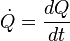$\dot{Q} = {dQ\over dt} \,\!$.

This is measured in watts. Heat flux is defined as rate of heat transfer per unit cross-sectional area, and is denoted q, resulting in units of watts per meter squared, though slightly different notation conventions can be used.

Heat is related to the internal energy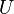$U$ of the system and work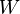$W$ done by the system by the first law of thermodynamics: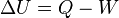$\Delta U = Q - W \$

which means that the energy of the system can change either via work or via heat. The transfer of heat to an ideal gas at constant pressure increases the internal energy and performs boundary work (that is, allows a control volume of gas to become larger or smaller), provided the volume is not constrained. Returning to the first law equation and separating the work term into two types, "boundary work" and "other" (such as shaft work performed by a compressor fan), yields the following:$\Delta U + W_{boundary} = Q - W_{other}\$

This combined quantity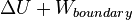$\Delta U + W_{boundary}$ is enthalpy,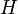$H$, one of the thermodynamic potentials. Both enthalpy,$H$, and internal energy,$U$ are state functions. State functions return to their initial values upon completion of each cycle in cyclic processes such as that of a heat engine. In contrast, neither$Q$ nor$W$ are properties of a system and need not sum to zero over the steps of a cycle. The infinitesimal expression for heat,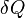$\delta Q$, forms an inexact differential for processes involving work. However, for processes involving no change in volume, applied magnetic field, or other external parameters,$\delta Q$, forms an exact differential. Likewise, for adiabatic processes (no heat transfer), the expression for work forms an exact differential, but for processes involving transfer of heat it forms an inexact differential.

The changes in enthalpy and internal energy can be related to the heat capacity of a gas at constant pressure and volume respectively. When there is no work, the heat,$Q$, required to change the temperature of a gas from an initial temperature, T0, to a final temperature, Tf depends on the relationship: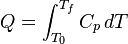$Q = \int_{T_0}^{T_f}C_p\,dT \,\!$

for constant pressure, whereas at constant volume: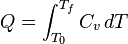$Q = \int_{T_0}^{T_f}C_v\,dT \,\!$

For incompressible substances, such as solids and liquids, there is no distinction among the two expressions, as they are nearly incompressible. Heat capacity is an extensive quantity and as such is dependent on the number of molecules in the system. It can be represented as the product of mass,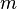$m$, and specific heat capacity,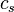$c_s \,\!$ according to:$C_p = mc_s \,\!$

or is dependent on the number of moles and the molar heat capacity,$c_n \,\!$ according to: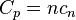$C_p = nc_n \,\!$

The molar and specific heat capacities are dependent upon the internal degrees of freedom of the system and not on any external properties, such as volume and number of molecules.

The specific heats of monatomic gases (for example, helium) are nearly constant with temperature. Diatomic gases such as hydrogen display some temperature dependence, and triatomic gases (forxample, carbon dioxide) still more.

In liquids at sufficiently low temperatures, quantum effects become significant. An example is the behavior of bosons such as helium-4. For such substances, the behavior of heat capacity with temperature is discontinuous at the Bose-Einstein condensation point.

The quantum behavior of solids is adequately characterized by the Debye model. At temperatures well below the characteristic Debye temperature of a solid lattice, its specific heat will be proportional to the cube of absolute temperature. A second, smaller term is needed to complete the expression for low-temperature metals having conduction electrons, an example of Fermi-Dirac statistics.

## Changes of phase

The boiling point of water, at sea level and normal atmospheric pressure and temperature, will always be at nearly 100°C, no matter how much heat is added. The extra heat changes the phase of the water from liquid into water vapor. The heat added to change the phase of a substance in this way is said to be "hidden," and thus it is called latent heat (from the Latin latere, meaning "to lie hidden"). Latent heat is the heat per unit mass necessary to change the state of a given substance, or: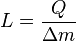$L = \frac{Q}{\Delta m} \,\!$

and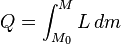$Q = \int_{M_0}^{M} L\,dm \,\!$

Note that as pressure increases, the L rises slightly. Here,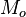$M_o$ is the amount of mass initially in the new phase, and M is the amount of mass that ends up in the new phase. Also, L generally does not depend on the amount of mass that changes phase, so the equation can normally be written: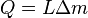$Q = L\Delta m \,\!$

Sometimes L can be time-dependent if pressure and volume are changing with time, so that the integral can be written as: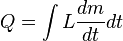$Q = \int L\frac{dm}{dt}dt \,\!$

## Heat transfer mechanisms

As mentioned previously, heat tends to move from a high temperature region to a low temperature region. This heat transfer may occur by the mechanisms conduction and radiation. In engineering, the term "convective heat transfer" is used to describe the combined effects of conduction and fluid flow and is regarded as a third mechanism of heat transfer.

### Conduction

Conduction is the most significant means of heat transfer in a solid. On a microscopic scale, conduction occurs as hot, rapidly moving or vibrating atoms and molecules interact with neighboring atoms and molecules, transferring some of their energy (heat) to these neighboring atoms.

### Convection

Convection is usually the dominant form of heat transfer in liquids and gases. This is a term used to characterize the combined effects of conduction and fluid flow. In convection, enthalpy transfer occurs by the movement of hot or cold portions of the fluid together with heat transfer by conduction. For example, when water is heated on a stove, hot water from the bottom of the pan rises, heating the water at the top of the pan. Two types of convection are commonly distinguished, "free convection," in which gravity and buoyancy forces drive the fluid movement, and "forced convection," where a fan, stirrer, or other means is used to move the fluid. Buoyant convection is heavily affected by gravity, and so does not occur in microgravity environments.